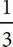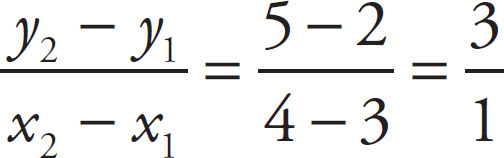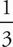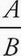# SAT Math Multiple Choice Question 47: Answer and Explanation

### Test Information

Question: 47

3. Line l contains points (3, 2) and (4, 5). If line m is perpendicular to line l, then which of the following could be the equation of line m ?

• A. x + 5y = 15
• B. x + 3y = 15
• C. 3x + y = 5
• D. -5x + y =B First, find the slope of line l by using the slope formula:. A line perpendicular to line l must have a slope that is the negative reciprocal of l's slope. So, its slope should be -. In the standard form of a line Ax + By = C, the slope is -. Only (B) has a slope of -. If you didn't remember the rule about the slope of perpendicular lines in standard form, you could have converted the answers to slope-intercept form and sketched out each of the lines to look for the answer that looked perpendicular to l.